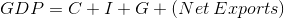# AP Macroeconomics : Real Output

## Example Questions

### Example Question #1 : Real Output

Which of the following is not a part of the business cycle?

Expansion

Plateau

Trough

Contraction

Plateau

Explanation:

The four phases of the business cycle are expansion, peak, contraction, and trough. A plateau is not one of these four phases.

### Example Question #1 : Fiscal Policy

How does an increase in imports affect a nation's GDP?

An increase in imports does not affect a nation's GDP.

An increase in imports raises a nation's GDP.

An increase in imports decreases a nation's GDP.

An increase in imports does not affect a nation's GDP.

Explanation:

Using the GDP equation, we see that any imports will be added to either Consumption or Investment, but will be subtracted from Net Exports by the same amount. This leads to a net change of zero.

### Example Question #1 : Real Output

A recessionary gap occurs when __________.

real output exceeds potential output

potential output exceeds real output

nominal output exceeds potential output

real output is equal to potential output

potential output exceeds real output

Explanation:

A recessionary gap is defined as a situation in which real output is below potential output. In other words, the economy could be producing more than it is.

The answer choice "real output exceeds potential output" is incorrect; it describes an inflationary gap.

The answer choice "nominal output exceeds potential output" is incorrect; inflationary and recessariony gaps refer to real output levels, not nominal levels.

### All AP Macroeconomics Resources